﻿ 利用1984~2022年日长实测值对其变化的时序分析和拟合研究文章快速检索 高级检索
 大地测量与地球动力学2023, Vol. 43Issue (4): 339-344  DOI: 10.14075/j.jgg.2023.04.002### 引用本文WEI Erhu, BAI Xiaoyu, LI Yanlin, et al. Study on Time Series Analysis and Fitting of Diurnal Length Variationwith Observations from 1984 to 2022[J]. Journal of Geodesy and Geodynamics, 2023, 43(4): 339-344.### Foundation support

National Natural Science Foundation of China, No.41874036; National Key Research and Development Program of China, No.2018YFC1503600.

### 第一作者简介

WEI Erhu, PhD, professor, PhD supervior, majors in space geodesy and navigation, E-mail: ehwei@sgg.whu.edu.cn.

### 文章历史

1. 武汉大学测绘学院，武汉市珞喻路129号，430079;
2. 中国地质大学(武汉)地理与信息工程学院，武汉市鲁磨路388号，430079;
3. 武汉大学卫星导航定位技术研究中心，武汉市珞喻路129号，430079

1 日长变化统计分析基础 1.1 地球自转速率变化

1.2 地球自转速率数据预处理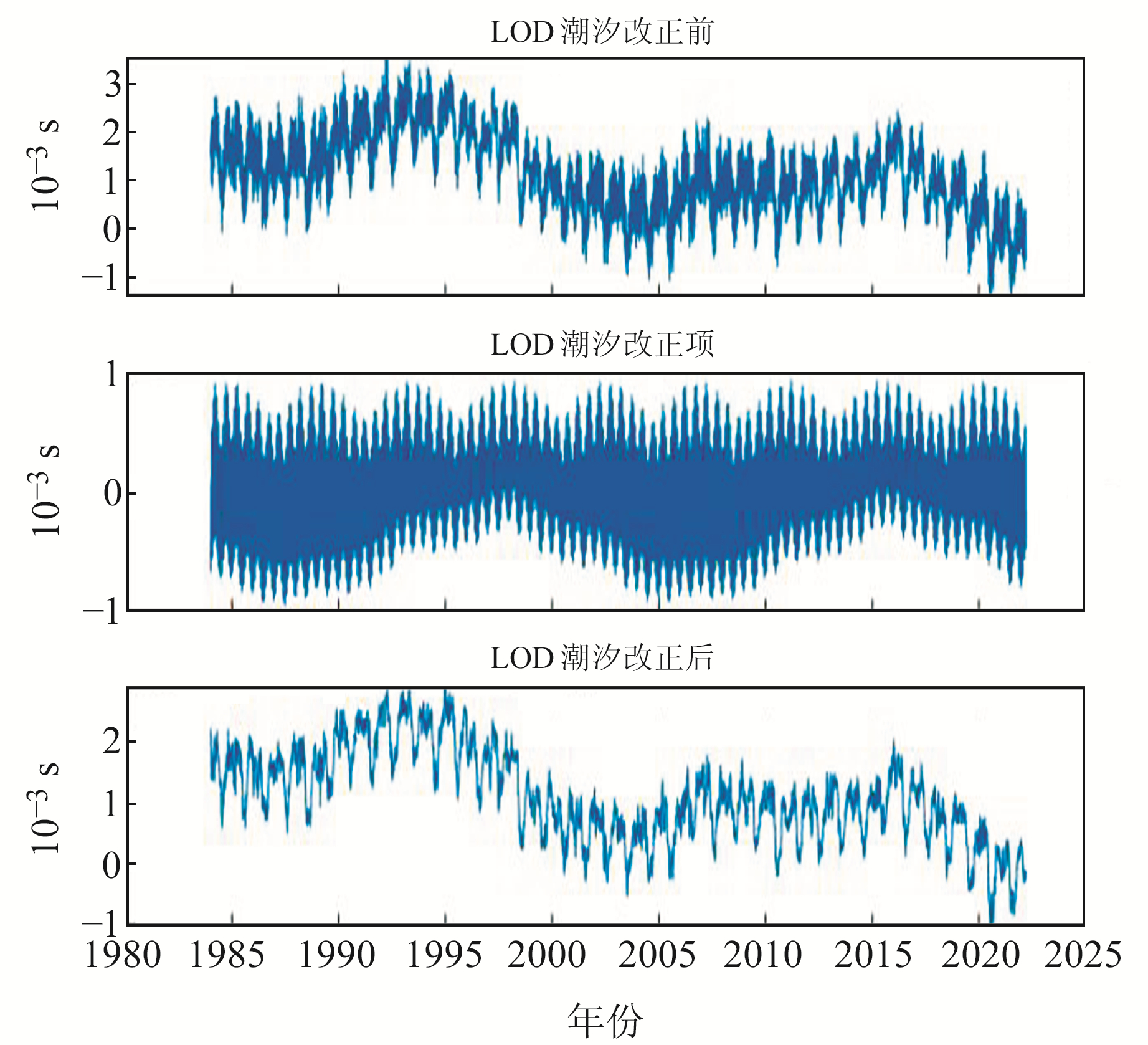图 1 LOD中的固体地球带谐潮汐改正 Fig. 1 Harmonic tidal correction of solid earth in LOD
2 日长变化不同周期提取方法对比 2.1 频谱分析及周期项提取

 $s_i=\sqrt{\left(\frac{2}{n} \sum\limits_{t=1}^{n-1} x_t \cos \frac{2 \pi i t}{n}\right)^2+\left(\frac{2}{n} \sum\limits_{t=1}^{n-1} x_t \sin \frac{2 \pi i t}{n}\right)^2}$ (1)

1) 对LOD序列进行傅里叶频谱分析，选出振幅最大的周期项；

2) 判断频谱分析结果中的最大振幅是否小于设定的阈值，若是则结束周期项提取，若否则进入步骤3)；

3) 用原始序列减去该振幅最大的周期项在原始序列中所占的部分，形成新的序列，再返回步骤1)对新序列进行傅里叶频谱分析。

 $\left\{\begin{array}{l} X_{t_{\mathrm{nc}}}=X_{t_{\mathrm{nc}-1}}-\left(c_{\mathrm{nc}} \cos \left(\frac{2 \pi t}{T_{\mathrm{nc}}}\right)+\right. \\\;\;\;\; \left.d_{\mathrm{nc}} \sin \left(\frac{2 \pi t}{T_{\mathrm{nc}}}\right)\right) \\ c_{\mathrm{nc}}=A_{\mathrm{nc}} \sin \left(\varphi_{\mathrm{nc}}\right) \\ d_{\mathrm{nc}}=A_{\mathrm{nc}} \cos \left(\varphi_{\mathrm{nc}}\right) \end{array}\right.$ (2)

2.2 拟合模型建立 2.2.1 最小二乘外推模型

 $\left\{\begin{array}{l} X_t=a+b t+\sum\limits_{i=1}^k\left(c_i \cos \left(\frac{2 \pi t}{T_i}\right)+\right. \\ \left.\quad d_i \sin \left(\frac{2 \pi t}{T_i}\right)\right)+\varepsilon_{\mathrm{LS}}(t) \\ c_i=A_i \sin \left(\varphi_i\right) \\ d_i=A_i \cos \left(\varphi_i\right) \end{array}\right.$ (3)

 \left\{ \begin{array}{l} \begin{aligned} & \left(\boldsymbol{\alpha}=\left[\begin{array}{lllllll} a & b & c_1 & d_1 & \cdots & c_k & d_k \end{array}\right]^{\mathrm{T}}\right. \\ & \boldsymbol{L}=\left[\begin{array}{llll} x_{t_1} & x_{t_2} & \cdots & x_{t_n} \end{array}\right]^{\mathrm{T}} \\ & \boldsymbol{A}=\left[\begin{array}{ccccccc} 1 & t_1 & \cos \left(\frac{2 \pi t_1}{T_1}\right) & \sin \left(\frac{2 \pi t_1}{T_1}\right) & \cdots & \cos \left(\frac{2 \pi t_1}{T_k}\right) & \sin \left(\frac{2 \pi t_1}{T_k}\right) \\ \vdots & \vdots & \vdots & \vdots & \vdots & \vdots & \vdots \\ 1 & t_n & \cos \left(\frac{2 \pi t_n}{T_1}\right) & \sin \left(\frac{2 \pi t_n}{T_1}\right) & \cdots & \cos \left(\frac{2 \pi t_n}{T_k}\right) & \sin \left(\frac{2 \pi t_n}{T_k}\right) \end{array}\right] \\ & \boldsymbol{\alpha}=\left(\boldsymbol{A}^{\mathrm{T}} \boldsymbol{E} \boldsymbol{A}\right)^{-1} \boldsymbol{A}^{\mathrm{T}} \boldsymbol{E} \boldsymbol{L} \\ & \end{aligned} \end{array} \right. (4)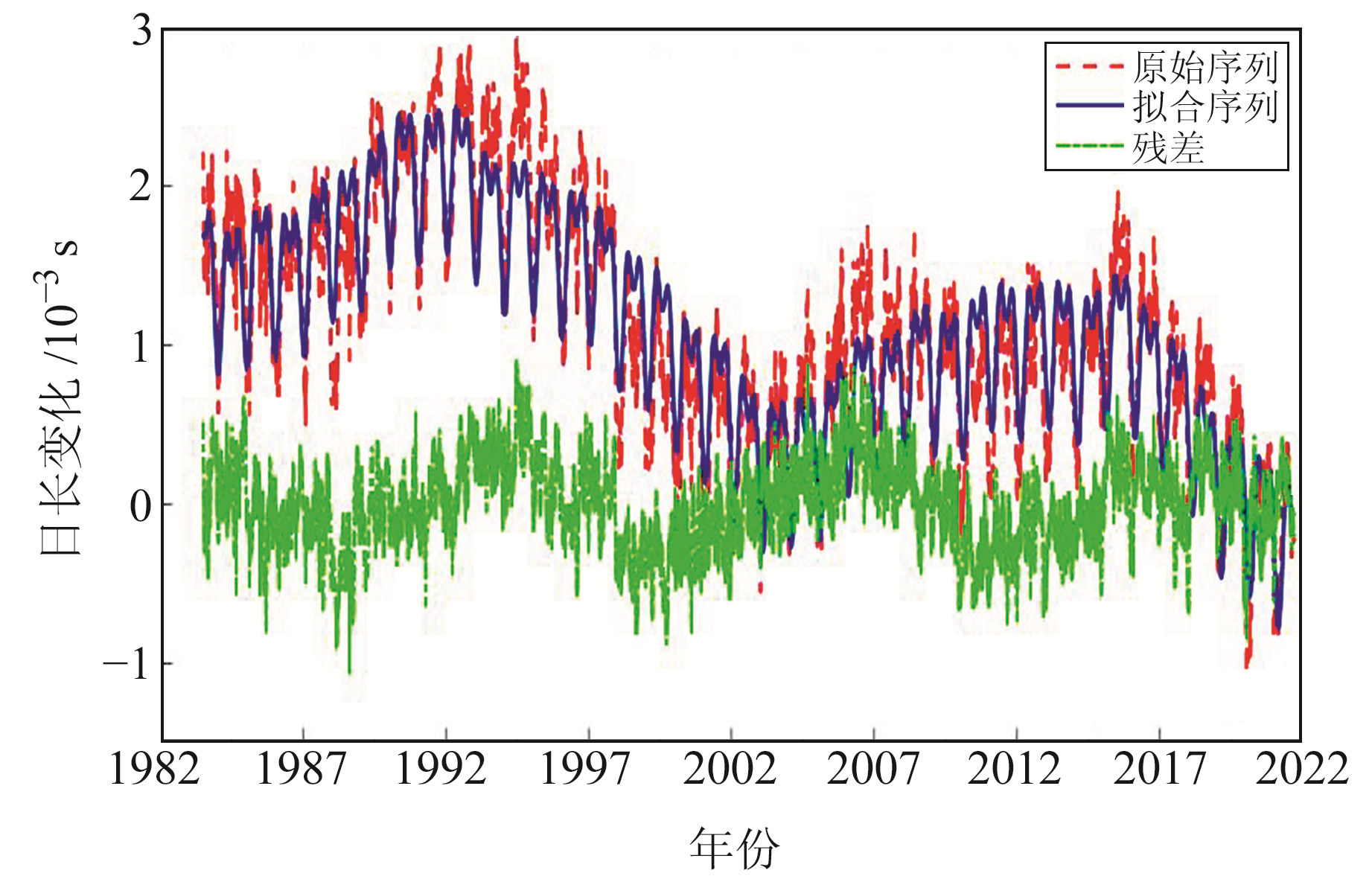图 2 LS外推模型拟合结果 Fig. 2 LS results based on fractional step periodic extraction表 1 周期提取结果(振幅大于等于0.03 ms) Tab. 1 Period extraction results(amplitude≥0.03 ms)
2.2.2 多项式曲线拟合模型

 $X_t=a+\sum\limits_{i=1}^m b_i t^i+\varepsilon_{\mathrm{PCF}}(t)$ (5)

 $\left\{\begin{array}{l} \boldsymbol{\alpha}=\left[\begin{array}{llll} a & b_1 & \cdots & b_m \end{array}\right]^{\mathrm{T}} \\ \boldsymbol{L}=\left[\begin{array}{llll} x_{t_1} & x_{t_2} & \cdots & x_{t_n} \end{array}\right]^{\mathrm{T}} \\ \boldsymbol{A}=\left[\begin{array}{cccc} 1 & t_1 & \cdots & t_1^m \\ \vdots & \vdots & \vdots & \vdots \\ 1 & t_n & \cdots & t_n^m \end{array}\right] \\ \boldsymbol{\alpha}=\left(\boldsymbol{A}^{\mathrm{T}} \boldsymbol{E} \boldsymbol{A}\right)^{-1} \boldsymbol{A}^{\mathrm{T}} \boldsymbol{E} \boldsymbol{L} \end{array}\right.$ (6)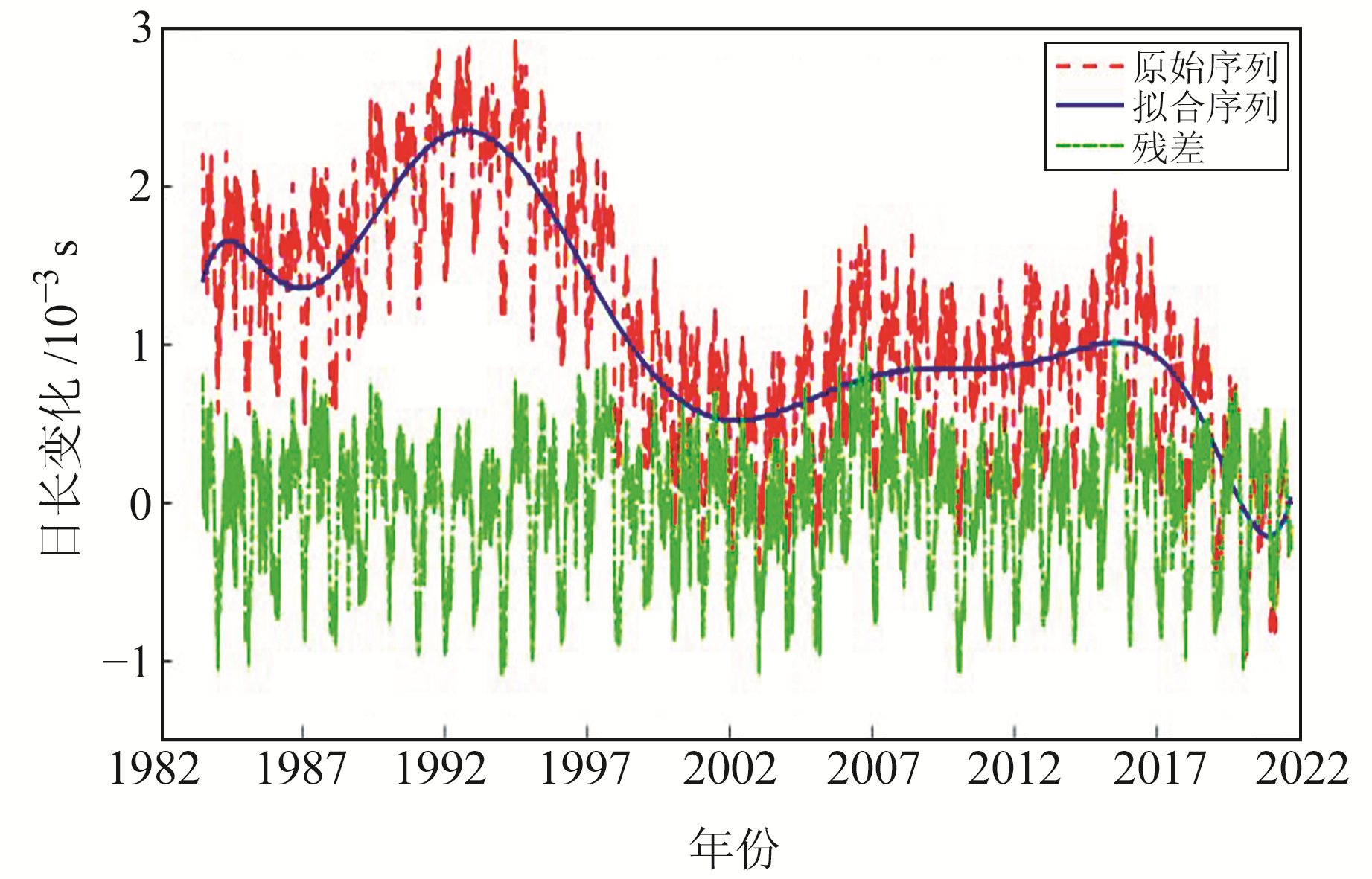图 3 10阶PCF模型拟合结果 Fig. 3 10 order PCF results

2.3 拟合模型结果

2.3.1 LS模型拟合效果分析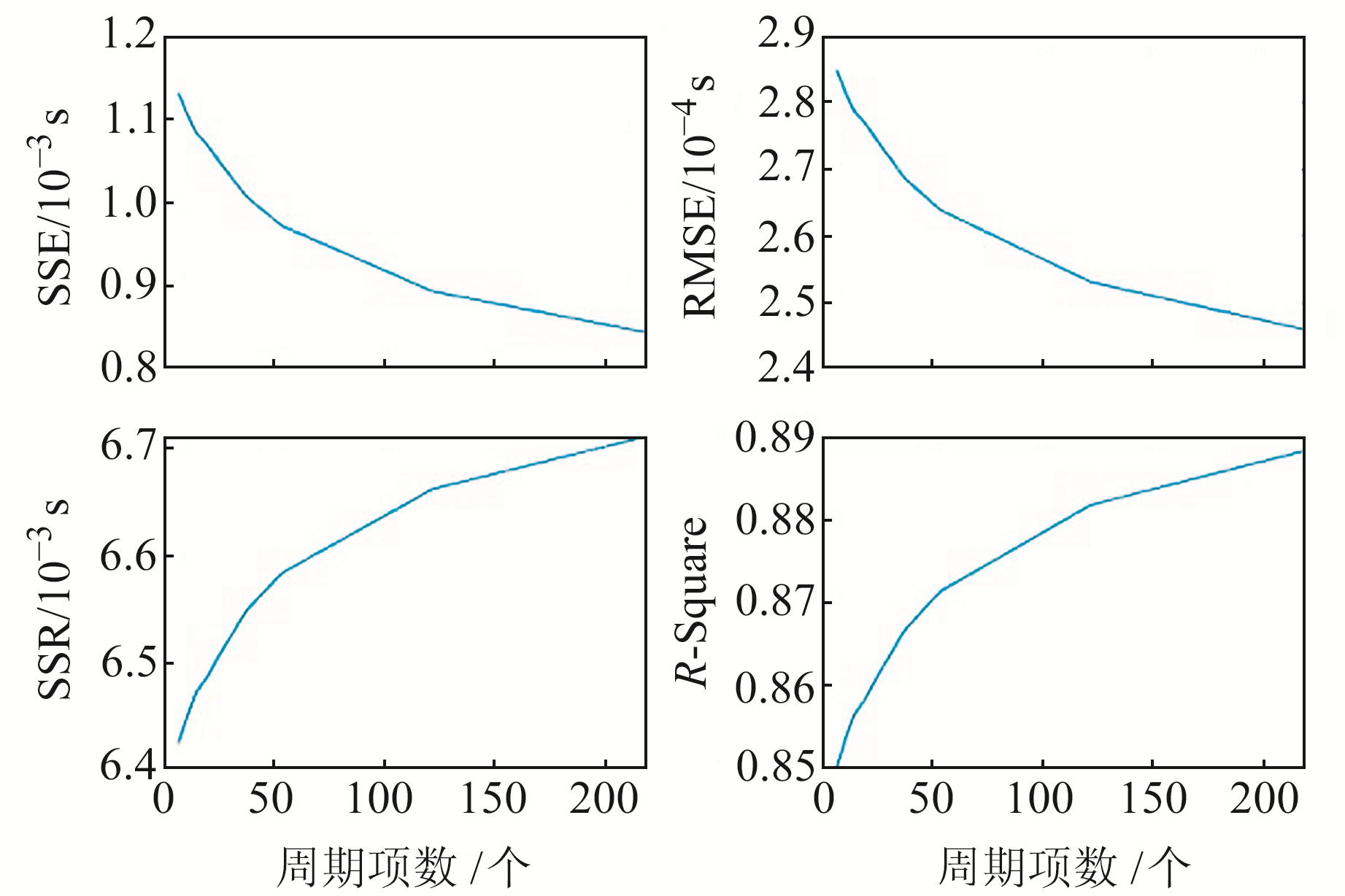图 4 不同周期项的LS外推模型拟合的各项指标 Fig. 4 Indexes of LS with different period terms

2.3.2 PCF模型拟合效果分析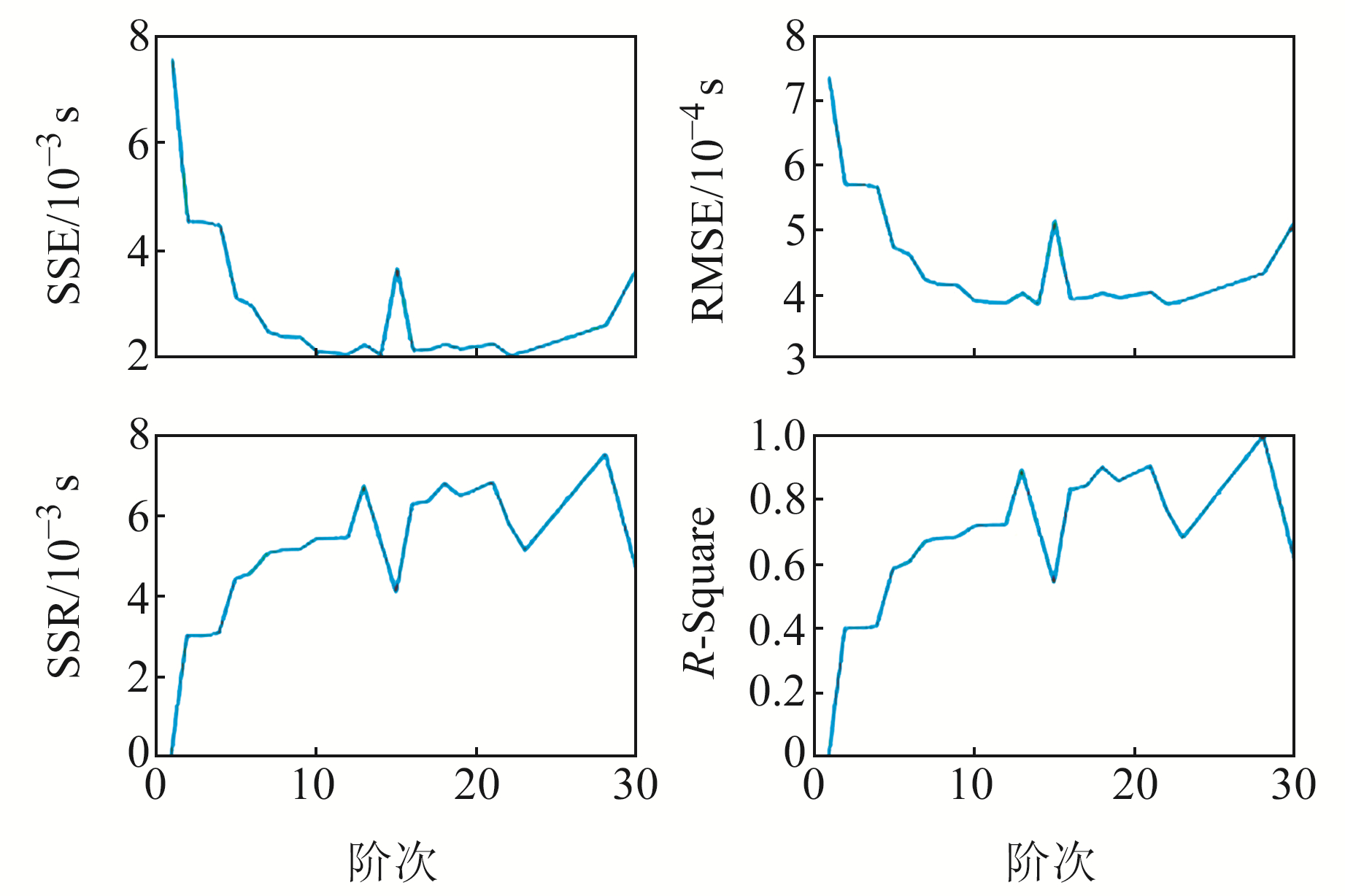图 5 不同阶次下PCF模型拟合的指标 Fig. 5 Index of PCF at different orders

3 日长变化序列拟合方法改进及预报

3.1 LOD序列拟合模型改进 3.1.1 LS+PCF模型拟合

LS+PCF模型是结合了LS外推模型中的常数项、趋势项、周期项以及PCF模型中的阶次项的一种拟合模型，其数学表达式为：

 $\left\{\begin{array}{l} X_t=a+\sum\limits_{i=1}^m\left(b_i t^i\right)+\sum\limits_{i=1}^k\left(c_i \cos \left(\frac{2 \pi t}{T_i}\right)+\right. \\ \left.\quad d_i \sin \left(\frac{2 \pi t}{T_i}\right)\right)+\varepsilon_{\mathrm{LS}+\mathrm{PCF}}(t) \\ c_i=A_i \sin \left(\varphi_i\right) \\ d_i=A_i \cos \left(\varphi_i\right) \end{array}\right.$ (7)

 $\left\{ \begin{array}{l} \boldsymbol{\alpha}=\left[\begin{array}{lllllllll} a & b_1 & \cdots & b_m & c_1 & d_1 & \cdots & c_k & d_k \end{array}\right]^{\mathrm{T}} \\ \boldsymbol{L}=\left[\begin{array}{llll} x_{t_1} & x_{t_2} & \cdots & x_{t_n} \end{array}\right]^{\mathrm{T}} \\ \boldsymbol{A}=\left[\begin{array}{ccccccccc} 1 & t_1 & \cdots & t_1^m & \cos \left(\frac{2 \pi t_1}{T_1}\right) & \sin \left(\frac{2 \pi t_1}{T_1}\right) & \cdots & \cos \left(\frac{2 \pi t_1}{T_k}\right) & \sin \left(\frac{2 \pi t_1}{T_k}\right) \\ \vdots & \vdots & \ddots & \vdots & \vdots & \vdots & \ddots & \vdots & \vdots \\ 1 & t_n & \cdots & t_n^m & \cos \left(\frac{2 \pi t_n}{T_1}\right) & \sin \left(\frac{2 \pi t_n}{T_1}\right) & \cdots & \cos \left(\frac{2 \pi t_n}{T_k}\right) & \sin \left(\frac{2 \pi t_n}{T_k}\right) \end{array}\right] \\ \boldsymbol{\alpha}=\left(\boldsymbol{A}^{\mathrm{T}} \boldsymbol{E} \boldsymbol{A}\right)^{-1} \boldsymbol{A}^{\mathrm{T}} \boldsymbol{E} \boldsymbol{L} \end{array} \right.$ (8)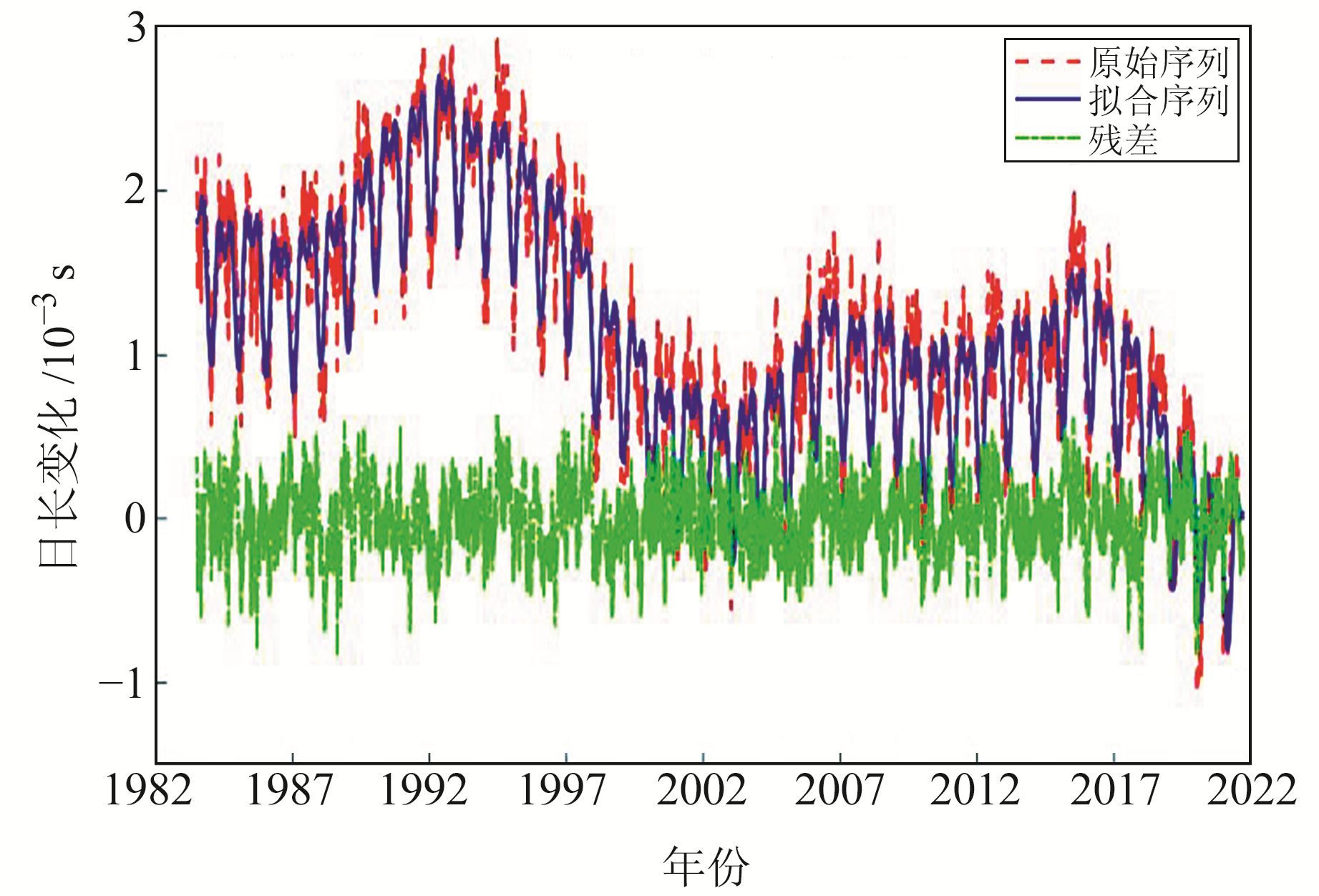图 6 LS+PCF模型拟合结果 Fig. 6 Fitting results of LS+PCF model

3.1.2 加权LS+PCF模型拟合

 $\boldsymbol{P}=\left[\begin{array}{cccc} \frac{\sigma_0^2}{\sigma_1^2} & 0 & 0 & 0 \\ 0 & \frac{\sigma_0^2}{\sigma_2^2} & 0 & 0 \\ 0 & 0 & \ddots & \vdots \\ 0 & 0 & \cdots & \frac{\sigma_0^2}{\sigma_n^2} \end{array}\right]$ (9)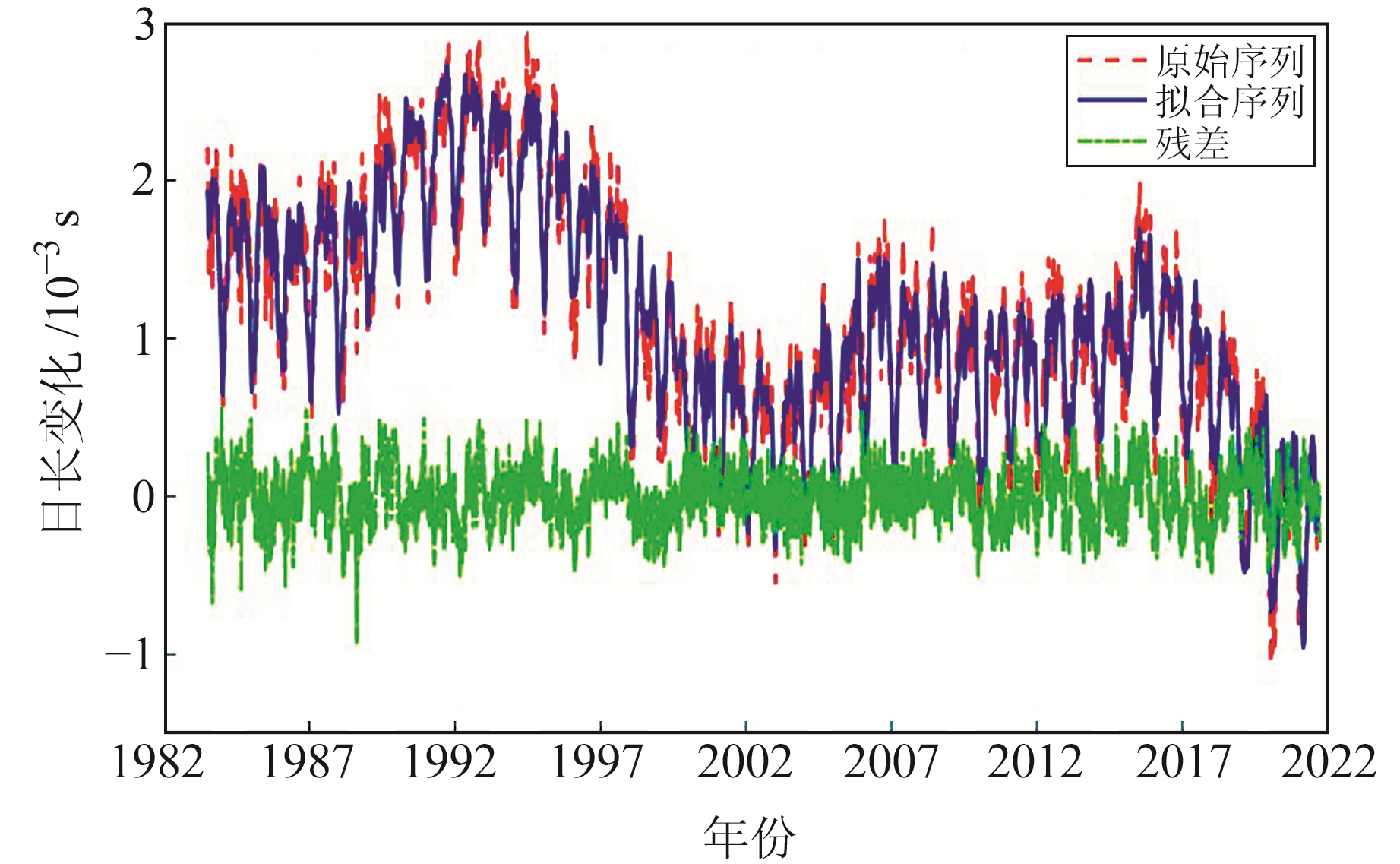图 7 加权LS+PCF模型拟合结果 Fig. 7 Fitting results of weighted LS+PCF model
3.1.3 改进后拟合模型效果分析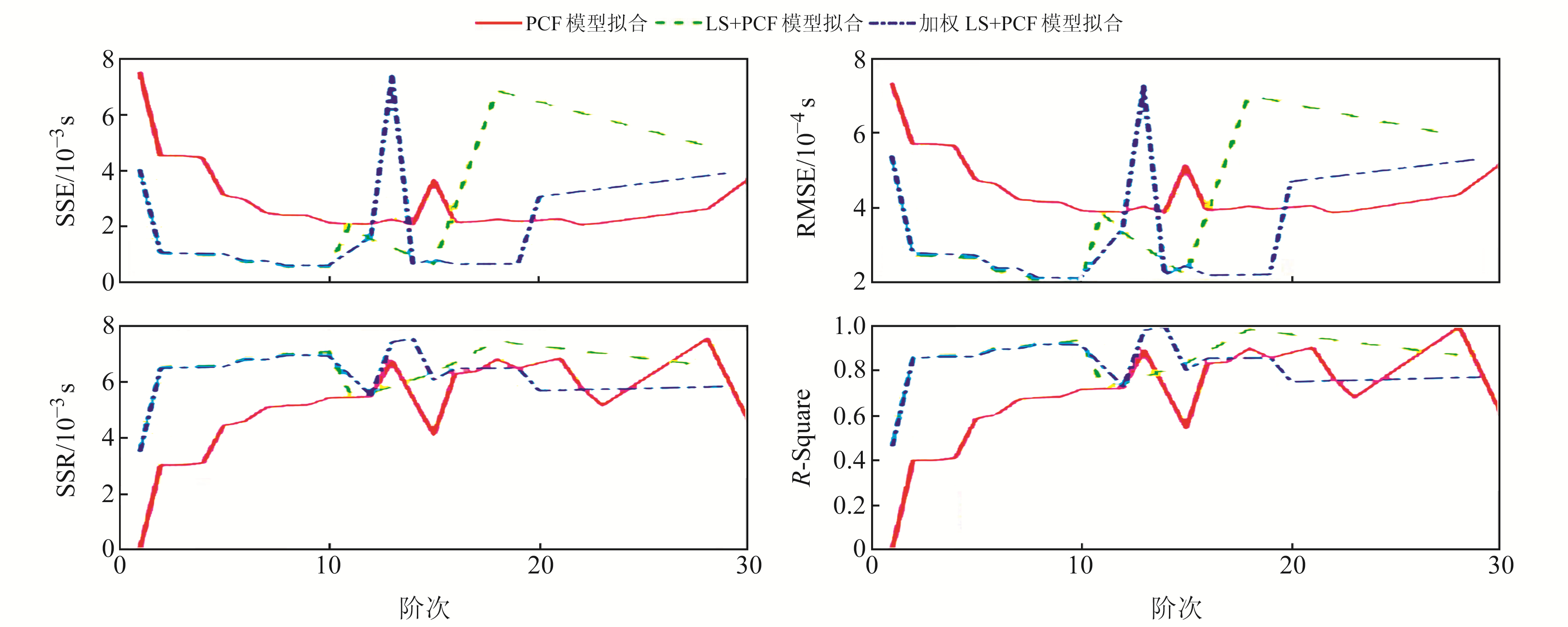图 8 不同阶次PCF模型拟合、LS+PCF模型拟合与加权LS+PCF模型拟合的各项指标 Fig. 8 Comparison of indexes of PCF, LS+PCF and weighted LS+PCF under different orders

3.2 LOD序列预报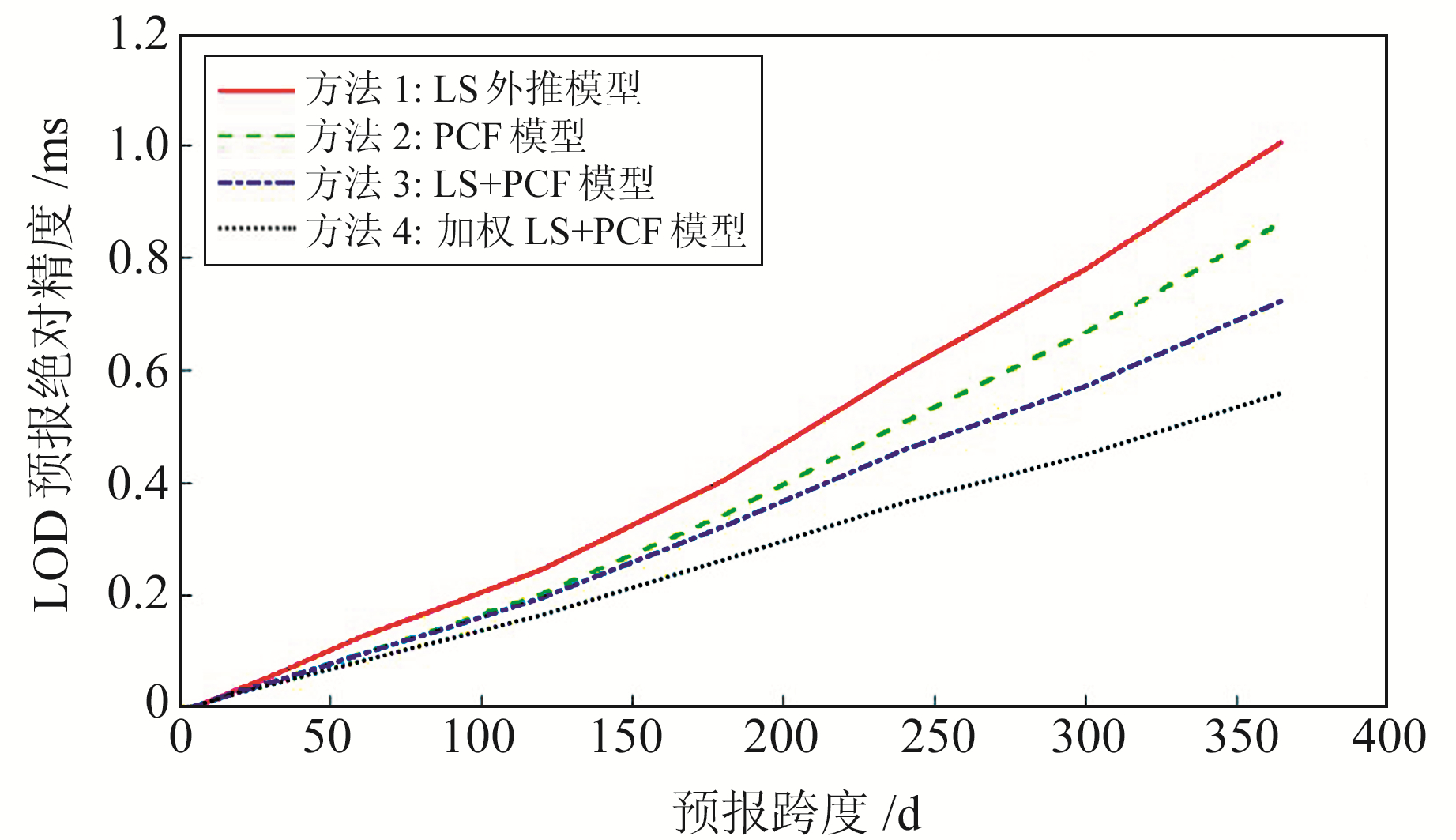图 9 4种模型的LOD预报绝对误差 Fig. 9 Absolute error of LOD prediction for 4 models

4 结语

  Zhu S Y. Prediction of Polar Motion[J]. Bulletin Géodésique, 1982, 56(3): 258-273 DOI:10.1007/BF02525586 (0)  Kosek W, Kalarus M, Niedzielski T. Forecasting of the Earth Orientation Parameters - Comparison of Different Algorithms[J]. Nagoya Journal of Medical Science, 2007(1): 155-158 (0)  Egger D. Neuronales Netz Pradiktion Von Erdrotation[J]. Allgemeine Vermessungs Nachrichten, 1992, 12(11): 247-258 (0)  Kalarus M. The Application of Artificial Neural Networks and Autoregressive Techniques for Earth Orientation Parameters Prediction[C]. European Geosciences Union General Assembly 2005, Vienna, Austria, 2005 (0)  Freedman A P, Steppe J A, Dickey J O, et al. The Short-Term Prediction of Universal Time and Length of Day using Atmospheric Angular Momentum[J]. Journal of Geophysical Research Solid Earth, 1994, 99(B4) (0)  雷雨. 地球自转参数高精度预报方法研究[D]. 北京: 中国科学院大学, 2016 (Lei Yu. Study on Methods for High Accuracy Prediction of Earth Rotation Parameters[D]. Beijing: University of Chinese Academy of Sciences, 2016) (0)  魏二虎, 刘学习, 孙浪浪, 等. 测站数目和观测弧段对GPS解算地球自转参数的影响分析[J]. 大地测量与地球动力学, 2017, 37(2): 187-191 (Wei Erhu, Liu Xuexi, Sun Langlang, et al. Analysis of the Influence of Number of Station and Observation Arcs to Earth Rotation Parameters by GPS[J]. Journal of Geodesy and Geodynamics, 2017, 37(2): 187-191) (0)  孙张振. 高精度地球自转参数预报模型与算法研究[D]. 济南: 山东大学, 2020 (Sun Zhangzhen. Research on the Models and Algorithms for High Accuracy Prediction of Faith Rotation Rotamters[D]. Jinan: Shandong University, 2020) (0)  魏二虎, 李岩林, 韩玉琴, 等. 地球自转速度变化与全球气温变化的相关性研究[J]. 测绘地理信息, 2021, 46(6): 1-7 (Wei Erhu, Li Yanlin, Han Yuqin, et al. Study on the Correlation between the Change of Earth Rotation Speed and the Change of Global Temperature[J]. Journal of Geomatics, 2021, 46(6): 1-7) (0)
Study on Time Series Analysis and Fitting of Diurnal Length Variationwith Observations from 1984 to 2022
WEI Erhu1BAI Xiaoyu2     LI Yanlin1     LIU Jingnan3
1. School of Geodesy and Geomatics, Wuhan University, 129 Luoyu Road, Wuhan 430079, China;
2. School of Geography and Information Engineering, China University of Geosciences, 388 Lumo Road, Wuhan 430079, China;
3. GNSS Research Centre, Wuhan University, 129 Luoyu Road, Wuhan 430079, China
Abstract: We use the measured LOD values of EOP 14 C04 from 1984 to 2022 released by the International Earth Rotation and Reference Systems Service(IERS) for spectrum analysis and period item extraction. We then use the method of least square extrapolation model combined with polynomial curve model to apply the extracted period term to the fitting of LOD sequence. The experimental results show that, compared with the single LS extrapolation model fitting, the RMSE of the fitting sequence decreases from 0.000 3 seconds to 0.000 1 seconds. The deterministic coefficient is increased from 0.8 to 0.97 by the new method. These results can provide reference for the prediction of LOD sequence.
Key words: length of day(LOD); spectrum analysis; periodic extraction; least square extrapolation model; polynomial curve fitting model